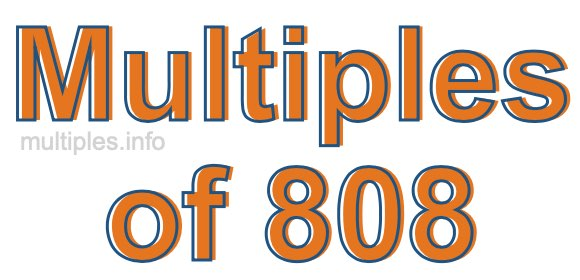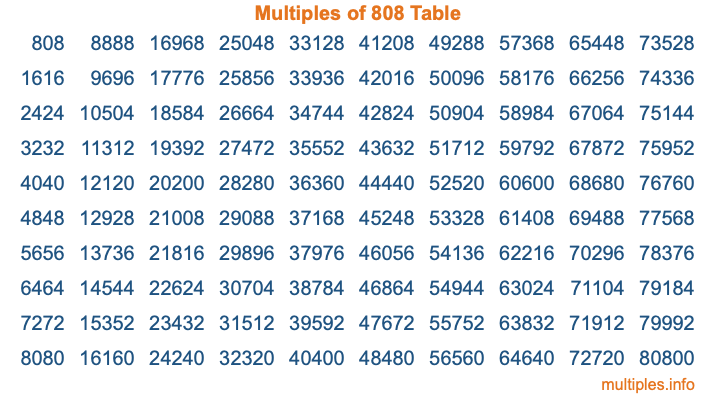Multiples of 808Welcome to the Multiples of 808 page. Here we will first teach you everything you will ever need to know about the multiples of 808, and then give you a study guide summary of everything we taught you to make sure you remember it all. Use this page to look up facts and learn information about the multiples of 808. This page will make you a multiples of eight hundred eight expert!

Definition of Multiples of 808
Multiples of 808 are all the numbers that when divided by 808 equal an integer. Each of the multiples of 808 are called a multiple. A multiple of 808 is created by multiplying 808 by an integer.

Therefore, to create a list of multiples of 808, you start with 1 multiplied by 808, then 2 multiplied by 808, then 3 multiplied by 808, and so on for as long as you want. Thus, the list of the first five multiples of 808 is 808, 1616, 2424, 3232, and 4040. To see a larger list of multiples of 808, see the printable image of Multiples of 808 further down on this page. We also have a category where you can choose any nth multiple of 808.

Multiples of 808 Checker
The Multiples of 808 Checker below checks to see if any number of your choice is a multiple of 808. In other words, it checks to see if there is any number (integer) that when multiplied by 808 will equal your number. To do that, we divide your number by 808. If the the quotient is an integer, then your number is a multiple of 808.

Is  a multiple of 808?

Least Common Multiple of 808 and ...
A Least Common Multiple (LCM) is the lowest multiple that two or more numbers have in common. This is also called the smallest common multiple or lowest common multiple and is useful to know when you are adding our subtracting fractions. Enter one or more numbers below (808 is already entered) to find the LCM.

Check out our LCM Calculator if you need more details about the Least Common Multiple or if you need the LCM for different numbers for adding and subtraction fractions.

nth Multiple of 808
As we stated above, 808 is the first multiple of 808, 1616 is the second multiple of 808, 2424 is the third multiple of 808, and so on. Enter a number below to find the nth multiple of 808.

th multiple of 808

Multiples of 808 vs Factors of 808
808 is a multiple of 808 and a factor of 808, but that is where the similarities end. All postive multiples of 808 are 808 or greater than 808. All positive factors of 808 are 808 or less than 808.

Below is the beginning list of multiples of 808 and the factors of 808 so you can compare:

Multiples of 808: 808, 1616, 2424, 3232, 4040, etc.

Factors of 808: 1, 2, 4, 8, 101, 202, 404, 808

As you can see, the multiples of 808 are all the numbers that you can divide by 808 to get a whole number. The factors of 808, on the other hand, are all the whole numbers that you can multiply by another whole number to get 808.

It's also interesting to note that if a number (x) is a factor of 808, then 808 will also be a multiple of that number (x).

Multiples of 808 vs Divisors of 808
The divisors of 808 are all the integers that 808 can be divided by evenly. Below is a list of the divisors of 808.

Divisors of 808: 1, 2, 4, 8, 101, 202, 404, 808

The interesting thing to note here is that if you take any multiple of 808 and divide it by a divisor of 808, you will see that the quotient is an integer.

Multiples of 808 Table
Below is an image of the first 100 multiples of 808 in a table. The table is in chronological order, column by column. The first column has the first ten multiples of 808, the second column has the next ten multiples of 808, and so on.The Multiples of 808 Table is also referred to as the 808 Times Table or Times Table of 808. You are welcome to print out our table for your studies.

Negative Multiples of 808
Although not often discussed or needed in math, it is worth mentioning that you can make a list of negative multiples of 808 by multiplying 808 by -1, then by -2, then by -3, and so on, to get the following list of negative multiples of 808:

-808, -1616, -2424, -3232, -4040, etc.

Multiples of 808 Summary
Below is a summary of important Multiples of 808 facts that we have discussed on this page. To retain the knowledge on this page, we recommend that you read through the summary and explain to yourself or a study partner why they hold true.

There are an infinite number of multiples of 808.

A multiple of 808 divided by 808 will equal a whole number.

808 divided by a factor of 808 equals a divisor of 808.

The nth multiple of 808 is n times 808.

The largest factor of 808 is equal to the first positive multiple of 808.

808 is a multiple of every factor of 808.

808 is a multiple of 808.

A multiple of 808 divided by a divisor of 808 equals an integer.

808 divided by a divisor of 808 equals a factor of 808.

Any integer times 808 will equal a multiple of 808.

Multiples of a Number
Here you can get the multiples of another number, all with the same attention to detail as we did for multiples of 808 on this page.

Multiples of
Multiples of 809
Did you find our page about multiples of eight hundred eight educational? Do you want more knowledge? Check out the multiples of the next number on our list!